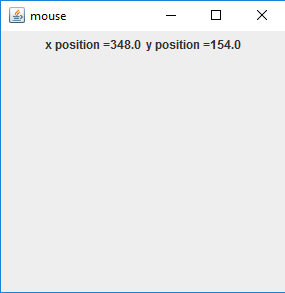Open in App
Not now

# Java AWT | MouseInfo and PointerInfo

• Last Updated : 31 Oct, 2019

MouseInfo and PointerInfo is a part of Java AWT. MouseInfo provides information about the location of the pointer and the number of buttons on the mouse. PointerInfo provides information returns the information about the location of the pointer and the graphics device.

#### Methods of MouseInfo

MethodExplanation
getNumberOfButtons()Returns the number of buttons on the mouse.
getPointerInfo()Returns a PointerInfo object that represents the current location and graphics device of the pointer.

#### Methods of PointerInfo

MethodExplanation
getDevice()Returns the graphics device on which mouse was present when the object was created .
getLocation()Returns a point object that gives the location of the mouse pointer.

• Example Program to find the number of buttons on the Mouse

 `// Java Program to find the number of``// buttons on the mouse``import` `java.awt.*;``import` `javax.swing.*;`` ` `class` `numberofbuttons {`` ` `    ``// Main Method``    ``public` `static` `void` `main(String args[])``    ``{``        ``int` `numberofbuttons;`` ` `        ``// number of buttons in the mouse``        ``numberofbuttons = MouseInfo.getNumberOfButtons();`` ` `        ``// print the number of buttons``        ``System.out.println(``"Number of buttons on the mouse ="` `                                           ``+ numberofbuttons);``    ``}``}`

Output:

```Number of buttons on the mouse = 5
```
• Example Program to show the Position of Mouse

 `// Java Program to show the``// position of mouse``import` `java.awt.*;``import` `java.awt.event.*;``import` `javax.swing.*;`` ` `class` `mouse ``extends` `JFrame {`` ` `    ``boolean` `b;`` ` `    ``// label``    ``JLabel l, l1;`` ` `    ``// Main Method``    ``public` `static` `void` `main(String args[])``    ``{`` ` `        ``// create object``        ``mouse m = ``new` `mouse();``    ``}`` ` `    ``// default constructor``    ``mouse()``    ``{``        ``super``(``"mouse"``);`` ` `        ``// create labels``        ``l = ``new` `JLabel(``""``);``        ``l1 = ``new` `JLabel(``""``);`` ` `        ``// create a panel``        ``JPanel p = ``new` `JPanel();`` ` `        ``// add labels to panel``        ``p.add(l);``        ``p.add(l1);`` ` `        ``add(p);``        ``show();``        ``setSize(``300``, ``300``);`` ` `        ``b = ``true``;``        ``execute();``    ``}`` ` `    ``public` `void` `execute()``    ``{``        ``while` `(b) {``            ``// get the pointer info object from the mouseInfo``            ``PointerInfo pi = MouseInfo.getPointerInfo();`` ` `            ``// get the location of mouse``            ``Point p = pi.getLocation();`` ` `            ``// set the text of labels``            ``l.setText(``"x position ="` `+ p.getX());``            ``l1.setText(``"y position ="` `+ p.getY());``        ``}``    ``}``}`

Output:References:

My Personal Notes arrow_drop_up